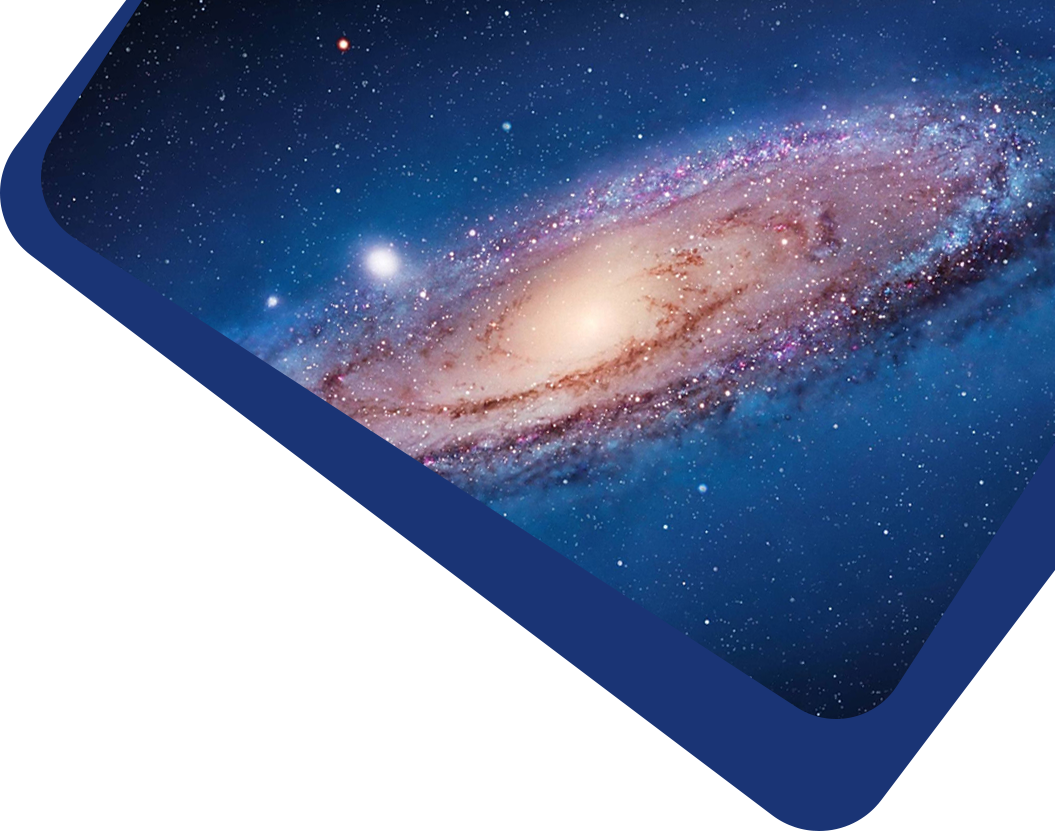Vol 21, No 2

## The moment generating function of pairwise velocity in the context of redshift space distortion

#### Jing-Wei Zhao, Jun-De Chen

Abstract

Abstract We study the connections between the pairwise velocity moment generating function $$G(k_{||}, \vec{r})$$ and redshift space distortion (RSD) modeling. Here $$k_{||}$$ is the Fourier wavevector parallel to the line of sight, and $$\vec{r}$$ is the pair separation vector. We demonstrate its usage by two examples. (1) Besides the known relations between $$G$$ and the RSD power spectrum (and the correlation function), we propose a new RSD statistics $$P^s (k_{||}, r_{\bot})$$ whose connection to $$G$$ is convenient to evaluate numerically. (2) We then develop a fast method to numerically evaluate $$G$$, and apply it to a high resolution N-body simulation. We find that $$G (\ln G)$$ shows complicated dependence on $$k_{||}$$ beyond the linear and quadratic dependencies. This not only shows inaccuracy in some existing models and identifies sources of inaccuracy but also provides possible ways of improving the RSD modeling. Consequently, more comprehensive investigations on $$G$$ are needed to fully explore the usage of $$G$$ in RSD modeling.

Keywords

Keywords cosmology: dark energy — dark matter — large-scale structure of universe

Full Text
Refbacks

• There are currently no refbacks.